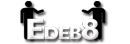Truncate HTML text with PHP

Normally on this blog I post updates relevant to edeb8. On this occasion, I want to share a particular function from the edeb8 source code that somebody might find useful out there.

Edeb8 requires me to truncate text containing HTML quite a bit to a specific number of characters. Usually I'll want well-formatted HTML at the end of it and for the character count to ignore the html tags, but I also needed the function to be reasonably small and customizable.

At first I tried a number of other code around the net but they turned out to be rather inaccurate and some led to very poorly formatted code. So I wrote my own function. Here it is in all its glory:

function edb8truncate(\$a,\$b=100,\$c='...',\$d=false,\$e=true){if(!\$e){if(\$d)\$f=substr(\$a,0,\$b);else \$f=substr(\$a,0,strrpos(substr(\$a,0,\$b),' '));if(strlen(\$f)<strlen(\$a))\$f=\$f.\$c;return \$f;}\$g=array();\$h=false;\$i=false;\$j=false;\$k="";\$l=0;\$m="";\$n=str_split(\$a);foreach(\$n as \$o=>\$p){if(\$p=="<"&&!\$h&&!\$j){\$h=true;if(\$m!=""){if(substr(\$m,-1)!="n"&&substr(\$m,-1)!="t"){\$m.="n";if(isset(\$n[\$o+1])){if(\$n[\$o+1]=="/")\$m.=str_repeat("t",count(\$g)-1);else \$m.=str_repeat("t",count(\$g));}else \$m.=str_repeat("t",count(\$g));}}}else if(\$h&&(\$p==">"||\$p==" ")){if(substr(\$k,-1)!="/")\$g[]=\$k;\$k="";\$h=false;if(\$p==" ")\$j=true;}else if(\$h&&\$p=="/"&&\$k==""){\$h=false;\$i=true;}else if(\$i&&(\$p==">"||\$p==" ")){if((\$q=array_search(\$k,\$g))!==false){unset(\$g[\$q]);}\$k="";\$i=false;}else if(\$h||\$i){\$k.=\$p;}else if(\$j&&\$p==">"){\$j=false;if(substr(\$m,-1)=="/")array_pop(\$g);}else if(!\$j){\$l=\$l+1;}\$g=array_diff(\$g,["hr","br","img","HR","BR","IMG"]);if(\$p!="t"&&\$p!="n")\$m.=\$p;if(\$p==">"){\$m.="n";if(isset(\$n[\$o+2])){if(\$n[\$o+1]=="<"&&\$n[\$o+2]=="/"&&count(\$g)>=1)\$m.=str_repeat("t",count(\$g)-1);else \$m.=str_repeat("t",count(\$g));}else \$m.=str_repeat("t",count(\$g));}if(!(\$h||\$i||\$j)&&\$l>\$b)break;}if(strlen(\$m)<strlen(\$a)){if(!\$d)\$m=substr(\$m,0,max(strrpos(\$m,' '),strrpos(\$m,'>')+1));\$m.=\$c;}\$g=array_reverse(\$g);foreach(\$g as \$q=>\$p){\$m.="n".str_repeat("t",count(\$g)-1);\$m.="</\$p>";unset(\$g[\$q]);}return "nn".\$m."nn";}

Some inaccuracies do exist in some conditions, but pretty much only for kinds of code you wouldn't want people to be able to put on your website anyway (like certain scripts). Combined with a decent sanitizer to ensure people don't post, say, tags with embedded scripts to your site, this is the best PHP function (in my experience) exists right now to truncate mixed text/html content people may post to your website.

How to use it

edb8truncate( \$content , \$length, [\$ending], [\$exact] , [\$consider-html] )

\$content - the mixed html/text content that you need to truncate
\$length - how many characters you need to truncate the content to (default: 100)
\$ending - if the user posted more they they're allowed to, then after truncation a text string is added to the content at the end (may be blank). What string is added may be changed with this parameter (default: "...")
\$exact - By default the function will truncate to the nearest word (or tag if there's a tag in the middle of a word right at the end of the truncation). Set this to true if you'd prefer to have it cut off in the middle of the word (default: false)
\$consider-html - set this to false if you've stripped any tags and just need a fast text truncation function with all the other parameters (default: true)

Returns: the truncated string

Note: this function is kinda slow. Most other attempts out there have used regular expressions - my approach is slower and, in my practical experience, considerably less prone to bugs (particularly when it comes to properly closing any remaining open tags).

Hope it's useful to somebody! Use it wherever you want.Comment posted by cperrot